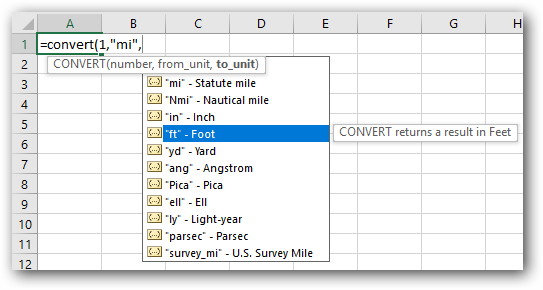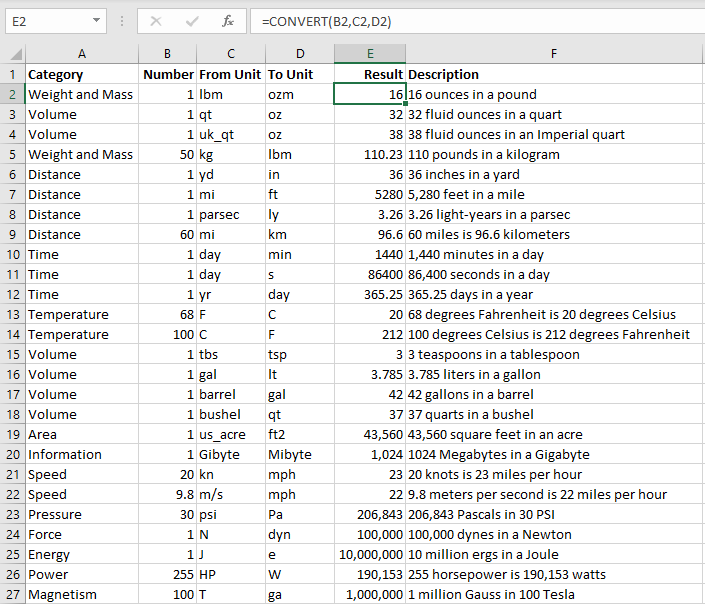Originally part of the Analysis ToolPak, CONVERT was added to the core Excel in 2007. Some improvements to the tooltip made it easier to use in Excel 2010. With the specific focus on scientific uses of Excel, the CONVERT function grew from 40 measurements to more than 100 in Excel 2013.

The CONVERT function will convert within any of these types of measurements: weight and mass, distance, time, temperature, volume, area, information, speed, pressure, force, energy, power, and magnetism. It’s great for converting from English to metric and also will help you convert within one measurement system, such as calculating feet to miles.

The syntax of CONVERT requires three arguments: =CONVERT(Number, From_unit, To_Unit). As you enter the formula, use the tooltip to select from a list. The list of units is particularly difficult to remember, especially those from the original version of CONVERT. For example, “oz” means fluid ounces and “ozm” means ounces of mass. And to be consistent with “ozm” as a unit of mass, the people who originally wrote CONVERT used “lbm” for pounds mass.

Another confusing set of units begins with “m.” While “m” is used for meter, “mn” means minute, “mi” means a statute mile, and “nmi” means a nautical mile. Unless you’re talking about speed, then use “kn” for knots and “mph” for miles per hour.

While the function was being rewritten for Excel 2013, the team at Microsoft allowed for some alternate abbreviations in the units of measure. For example, “min” can now be used for minutes in addition to “mn.” You can use “sec” or “s” for seconds. The old “pc” for parsec can now be written as “parsec.”

Plus, the units have to be wrapped in quotation marks if you’re typing them directly into the formula. By using the tooltip to select the unit of measurement, you’ll automatically get =CONVERT(1,“mi”,“ft”) instead of the error-producing =CONVERT(1,mi,ft).The codes for the units of measure are case-sensitive. Offering a castaway on a deserted island a “pl” of water vs. a “PL” of water is the difference between a drop of water and enough water to sink the island.

### THE TOOLTIP IS INCOMPLETE

While the addition of the tooltip in Excel 2010 went a long way toward making the function easier to use, it doesn’t handle the complete set of prefixes. As mentioned before, “m” means meter, and the tooltip offers this unit. Yet there are 20 prefixes that can be used before the “m,” and none of these are in the tooltip. Some examples:

• “mm” is millimeters
• “cm” is centimeters
• “km” is kilometers (or 1,000 meters)
• “Mm” is megameters (or 1 million meters)

There are prefixes for larger numbers (G, T, P, E, Z, and Y for giga, tera, peta, exa, zetta, and yotta, respectively). There also are prefixes for smaller fractions (u, n, p, f, a, z, and y for micro, nano, pico, femto, atto, zepto, and yocto).

Information measurements have a different set of prefixes. While the tooltip offers “bit” and “byte,” you can prefix either with “ki” for 2^10, “Mi” for 2^20, “Gi” for 2^30, as well as “Ti,” “Pi,” “Ei,” “Zi,” and “Yi.”

The complete list of prefixes can be found in the Excel Help topic for the formula. This is one case where you might need to visit the Help topic frequently to learn all of the units of measure and valid prefixes.

### REFERRING TO MEASUREMENT UNITS IN CELLS

If you build a formula that refers to units of measurement entered into other cells, you don’t have to wrap those cells in quotation marks. This is how I prefer to use the CONVERT function. In the figure, the formula =CONVERT(B2,C2,D2) converts the numbers in column B from the units in C to the units in D.As you can see, the CONVERT function handles almost every measurement conversion you might need. There are other functions in Excel that do conversions: The BASE function converts between binary, decimal, and hexadecimal. The ROMAN and ARABIC functions convert to Roman numerals and back. The new Stocks Data Type offers a formula to do currency conversions. But for most common conversions, CONVERT is the solution.

### SF SAYS

The CONVERT function handles measures both large and small, from square light-years to cubic picas.